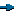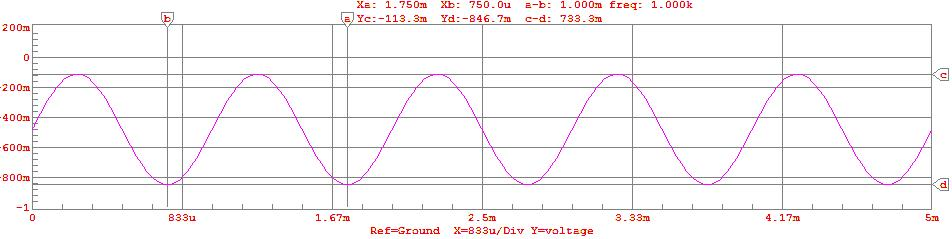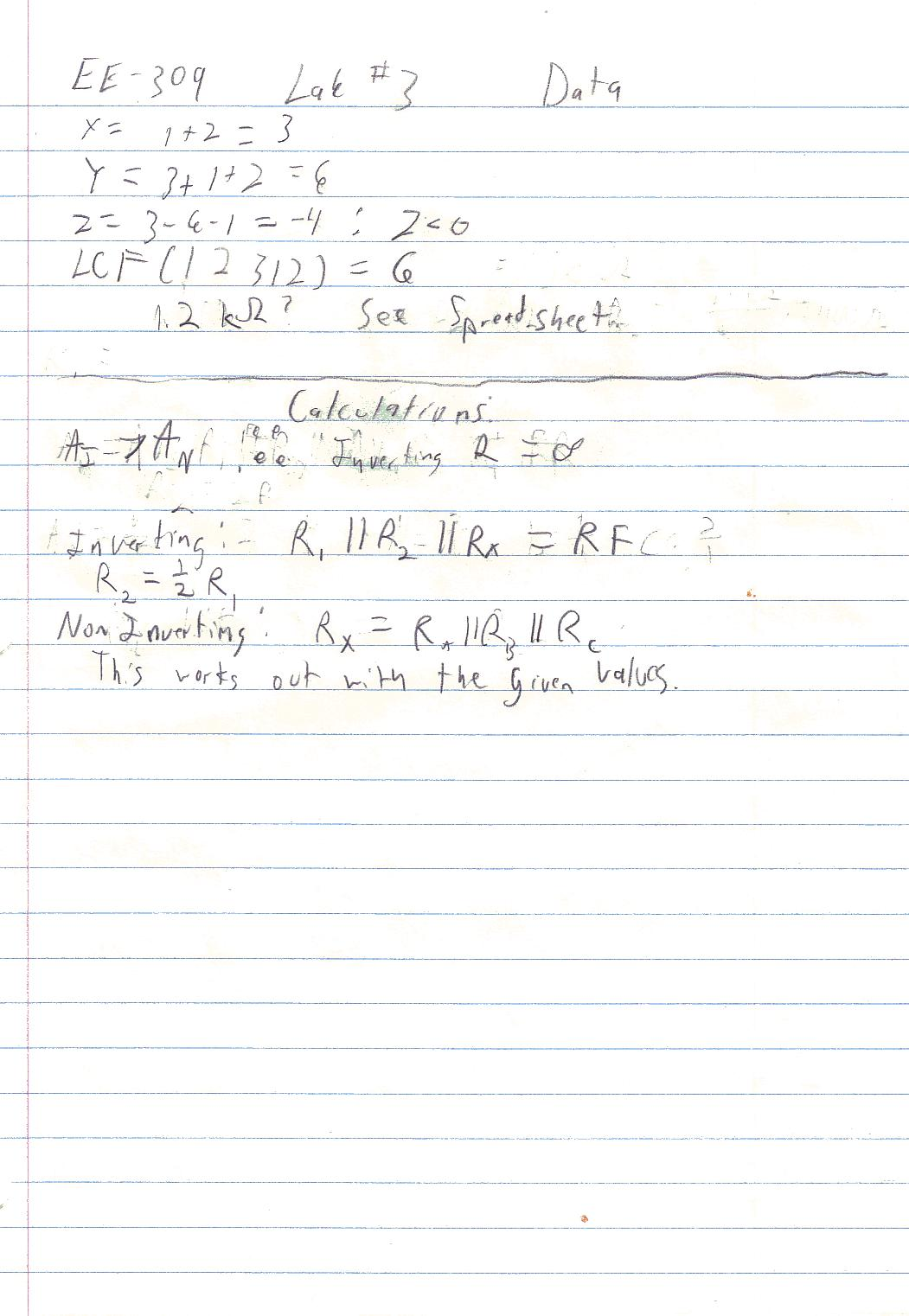EE - 309 / E01 Fall 2002

Circuit Design and Simulation

Professor: Ho

Submitted By:

Andrew Buettner

Lab #3: Op-Amp With Multiple Inputs

Thursday, March 06, 2003

1) Cover Page 1

3) Objective 3

4) Components Used 3

5) Procedures 3

6) Lab Data / Results 3

1) Diagram 1 3

2) Table 1 3

3) Diagram 2 4

4) Diagram 3 4

5) Diagram 4 4

7 Answers to Lab Questions 4

7) Conclusions 5

8) Attachments 5Objective

The objective of this lab is to create an amplifier with multiple inverting, and non inverting inputs. This will be accomplished by using a single 741 style op-amp. Then measurements will be bade on the simulated circuit and compared to expected, calculate values.Components Used

1) PC with CircuitMaker® installerProcedures

1) Design a multi-input amplifier / summer that follows the following equation: Vo = V1 + 2V2 - 3Va - Vb - 2Vc

2) Simulate the circuit using all 1V inputs.

3) Repeat step 2 using a 1Vp-p sine wave at 1KHz

4) Repeat step 2 using a 1Vp-p AM wave form with a carrier frequency of 10KHz and modulation of 1KHz.

5) Repeat step 2 using a 1Vp-p FM wave with the same characteristics as the AM wave.Lab Data / Results

1) Diagram 1: Summer / Amplifier Schematic2) Table 1: Results from DC test

 DC Voltage Output: -107.2mV

3) Diagram 2: Result from sinewave test4) Diagram 3: Results from AM test5) Diagram 4: Results from FM testAnswers to Lab Questions

1) Q: Derive Phil Vrbancic's theorem.

A: See AttachmentsConclusions

This lab has demonstrated the basics of a summer / amplifier. My answer to the post lab question may not be completely correct because I mostly guessed as to the Rx relationship. However, the system makes sense when considering the principle of inverting, and non-inverting configurations.Attachments

1. Original lab handout

2. Original lab data

3. Calculation